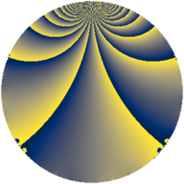# Properties

 Label 441.1.mLevel $441$ Weight $1$ Character orbit 441.m Rep. character $\chi_{441}(19,\cdot)$ Character field $\Q(\zeta_{6})$ Dimension $2$ Newform subspaces $1$ Sturm bound $56$ Trace bound $0$

# Related objects

## Defining parameters

 Level: $$N$$ $$=$$ $$441 = 3^{2} \cdot 7^{2}$$ Weight: $$k$$ $$=$$ $$1$$ Character orbit: $$[\chi]$$ $$=$$ 441.m (of order $$6$$ and degree $$2$$) Character conductor: $$\operatorname{cond}(\chi)$$ $$=$$ $$7$$ Character field: $$\Q(\zeta_{6})$$ Newform subspaces: $$1$$ Sturm bound: $$56$$ Trace bound: $$0$$

## Dimensions

The following table gives the dimensions of various subspaces of $$M_{1}(441, [\chi])$$.

Total New Old
Modular forms 34 6 28
Cusp forms 2 2 0
Eisenstein series 32 4 28

The following table gives the dimensions of subspaces with specified projective image type.

$$D_n$$ $$A_4$$ $$S_4$$ $$A_5$$
Dimension 2 0 0 0

## Trace form

 $$2q + q^{4} + O(q^{10})$$ $$2q + q^{4} - q^{16} - q^{25} + 2q^{37} - 4q^{43} - 2q^{64} - 2q^{67} - 2q^{79} + O(q^{100})$$

## Decomposition of $$S_{1}^{\mathrm{new}}(441, [\chi])$$ into newform subspaces

Label Dim. $$A$$ Field Image CM RM Traces $q$-expansion
$$a_2$$ $$a_3$$ $$a_5$$ $$a_7$$
441.1.m.a $$2$$ $$0.220$$ $$\Q(\sqrt{-3})$$ $$D_{2}$$ $$\Q(\sqrt{-3})$$, $$\Q(\sqrt{-7})$$ $$\Q(\sqrt{21})$$ $$0$$ $$0$$ $$0$$ $$0$$ $$q-\zeta_{6}^{2}q^{4}-\zeta_{6}q^{16}+\zeta_{6}^{2}q^{25}+\zeta_{6}q^{37}+\cdots$$